2013-09-06 22:57:46 kezunhai 阅读数 8940
• ###### MATLAB图像处理

全面系统的学习MATLAB在图像处理中的应用

19901 人正在学习 去看看 魏伟

论文转载请注明出处：http://blog.csdn.net/kezunhai

在前面的博文中，介绍了Moravec算子，并对moravec算子的不足也进行了简单的描述，具体请参考：图像处理特征算子系列之Moravec算子（一）。Harris算子针对Moravec算子的不足，提出了以下的改进：

1）Moravec算子各向异性响应（Anisotropic Response of Operator

Moravec算子仅仅在8个方向（水平、垂直和四个对角方向）计算灰度变化，为了对其扩展，有必要设计一个可以在任何方向对灰度变化进行测度的函数。1988年，Harris和Stephen通过对Moravec算子进行展开，推导得到了Plessey算子，也即Harris算子。

我们先来看看与Harris相关的背景知识。通常，Prewitt算子被用来对图像的梯度进行近似。然而，在实际应用中，一阶梯度通过下图中的公式来进行近似：通过上面的分析，灰度的变化可以表示为图像梯度的函数，公式表示如下：到这里，大家非常明了：上式可以对moravec算子中的灰度变化计算进行精确的逼近。但是又与Moravec算子中灰度变化不同的是通过合理的选择（u，v）可以对任何方向的灰度变化进行测度。

2）噪声响应  （Noise Response

在Moravec算子中，滑动窗采用的是方形的（square window），方形窗使得不同方向上的中心像素与边界像素的欧式距离是变化的。为了克服这个问题，Harris&Stephen提出只需将方向窗改成圆窗（circle window）。同时，窗中的每个像素是同等地位的，理论上应该是离中心越近的权重越大，而离中心越远，权重越小，因此我们加入高斯权重。因此，灰度变化的新测度方式可以通过下图来表示：通过公式表示如下：3）边缘的强响应（Large Response of Edge

因为Moravec算子在边缘处很容易出现误检，Harris&Stephen通过考虑不同方向的灰度度量形成新的角度性测度（cornerness measure）。接着，我们对上面的式子进行变换，如下式：Harris&Stephen同时也注意到，上式可以写成：同样，与Moravec算子一样，我们再来看下面的四张张图：Harris&Stephen提出下面的角点性测度（cornerness measure）

:k一般取值04~0.6。

最后，我们来总结下Harris算子的计算步骤：

（ 1）对每一个像素计算自相关矩阵M（2）构造角点性映射图（Construct cornerness map）（3）阈值化，对得到的C(x,y)进行阈值

（4）非极大值抑制

总结：Harris算子针对Moravec算子的不足进行了改进，提高了特征点的检测率以及Repeatability。但是，Harris算子计算量大，对尺度很敏感，不具有尺度不变形；另外Harris对特征点的定位也不是很精确，而且Harris也是各向异性的，对噪声敏感。

2016-01-07 15:06:30 lanchunhui 阅读数 2123
• ###### MATLAB图像处理

全面系统的学习MATLAB在图像处理中的应用

19901 人正在学习 去看看 魏伟
• time domain：时域， 如speech signal；spatial domain： 空域， 如image
• transform domain：变换域， 如傅里叶域的傅里叶系数，小波域的小波系数
• corpus：预料库（文本建模基本术语），一般由多篇文档构成的可称为预料库，记一篇文档 d=w⃗ =(w1,w2,,wn)$d=\vec w=(w_1,w_2,\ldots,w_n)$（由 n$n$个单词构成）；
• signal corruption：noise, missing data and outliers.
• on many benchmark visual datasets for different tasks
• performance metrics（性能度量）
• streaming workflows with pipelines

## 1. 计算机视觉的挑战

1. viewpoint variation：视角
2. scale variation：尺度
3. deformation：变形
4. occlusion：遮挡
5. illumination conditions：照明
6. background clutter：前景背景混淆
7. Intra-class variation：类内的差异## 2. DC（direct current）

• 一个图像块的 DC，通常意味着其均值。还记得二维傅里叶变换，

F(u,v)=xyf(x,y)ei2π(ux/M+vy/N)

其在(0, 0)（频率原点），

F(0,0)=xyf(x,y)

就对应着直流分量。

## 3. Population Sparsity（种群稀疏） vs Lifetime Sparsity（存在稀疏）

Fn×m=Wn×dXd×m

• d$d$：表示原有的特征空间
• n$n$：变换后的特征空间
• m$m$：样本的数目
• F$F$：特征矩阵（Feature Matrix）

## 4. High Dispersal（高分散性）

2019-03-19 23:12:23 weixin_43837871 阅读数 179
• ###### MATLAB图像处理

全面系统的学习MATLAB在图像处理中的应用

19901 人正在学习 去看看 魏伟

# 图像映射

图像映射就是图像之间的变换，加上使用一些计算变换的方法。可以实现图像扭曲变形和图像配准，适用于全景拼接。普遍变换方法有单应性变换、仿射变换、阿尔法通道等等。图像的映射类型有：平移、旋转、仿射、透视映射、尺度变换，不同的类型对应不一样的方法。经过这些处理就可以达到自己想要实现的映射效果。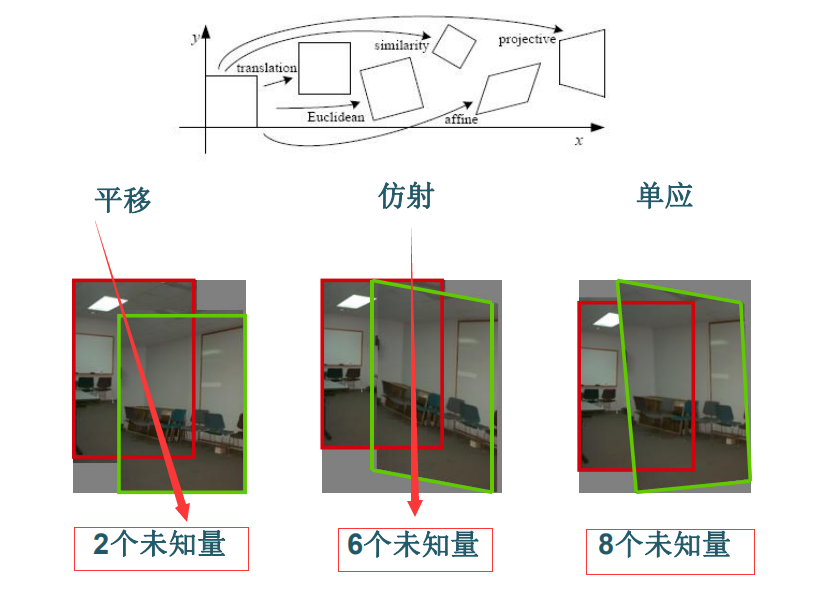## （一）原理解析

### （1）单应性变换（homography）

矩阵的一个重要作用是将空间中的点变换到另一个空间中。比较形像直观地理解的方法就是图像变换，图像变换的方法很多，单应性变换是其中一种方法，单应性变换会涉及到单应性矩阵。单应性变换的目标是通过给定的几个点（通常是4对点）来得到单应性矩阵。以下为单应性矩阵的推导过程。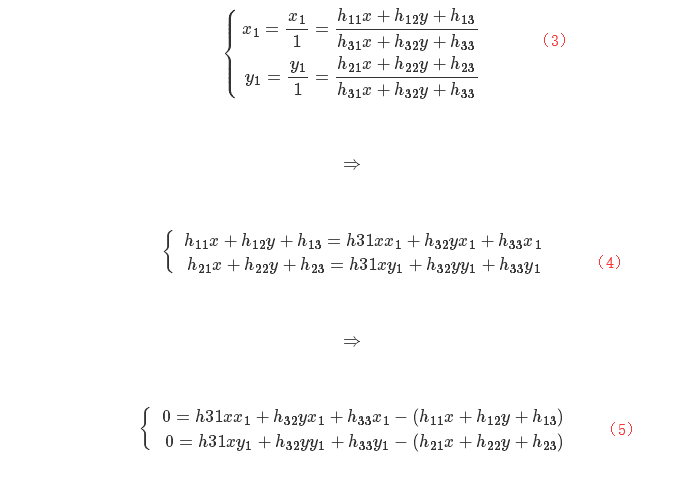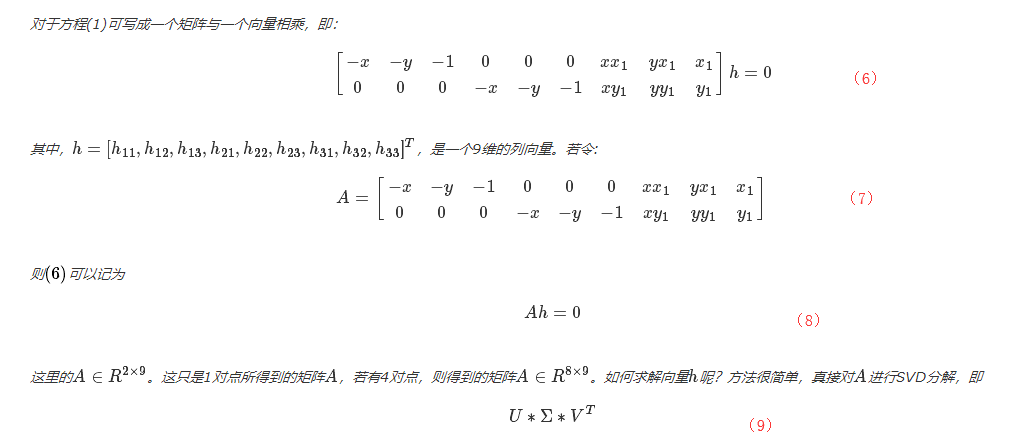### （2）仿射变换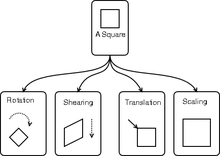## （二）仿射变换将一幅图像放置到另外一幅图像中

仿射扭曲im1到im3的例子：

 # -*- coding: utf-8 -*-
from PCV.geometry import warp, homography
from PIL import  Image
from pylab import *
from scipy import ndimage

# example of affine warp of im1 onto im2

im1 = array(Image.open('E.jpg').convert('L'))
im2 = array(Image.open('G.jpg').convert('L'))
# set to points
#tp = array([[120,260,260,120],[16,16,305,305],[1,1,1,1]])
tp = array([[600,2250,2550,600],[500,500,2400,2400],[1,1,1,1]])
im3 = warp.image_in_image(im1,im2,tp)
figure()
gray()
subplot(141)
axis('off')
imshow(im1)
subplot(142)
axis('off')
imshow(im2)
subplot(143)
axis('off')
imshow(im3)

# set from points to corners of im1
m,n = im1.shape[:2]
fp = array([[0,m,m,0],[0,0,n,n],[1,1,1,1]])
# first triangle
tp2 = tp[:,:3]
fp2 = fp[:,:3]
# compute H
H = homography.Haffine_from_points(tp2,fp2)
im1_t = ndimage.affine_transform(im1,H[:2,:2],
(H[0,2],H[1,2]),im2.shape[:2])
# alpha for triangle
alpha = warp.alpha_for_triangle(tp2,im2.shape,im2.shape)
im3 = (1-alpha)*im2 + alpha*im1_t
# second triangle
tp2 = tp[:,[0,2,3]]
fp2 = fp[:,[0,2,3]]
# compute H
H = homography.Haffine_from_points(tp2,fp2)
im1_t = ndimage.affine_transform(im1,H[:2,:2],
(H[0,2],H[1,2]),im2.shape[:2])
# alpha for triangle
alpha = warp.alpha_for_triangle(tp2,im2.shape,im2.shape)
im4 = (1-alpha)*im3 + alpha*im1_t
subplot(144)
imshow(im4)
axis('off')
show()

warp.py.

from scipy.spatial import Delaunay
from scipy import ndimage
from pylab import *
from numpy import *

from PCV.geometry import homography

def image_in_image(im1,im2,tp):
""" Put im1 in im2 with an affine transformation
such that corners are as close to tp as possible.
tp are homogeneous and counter-clockwise from top left. """

# points to warp from
m,n = im1.shape[:2]
fp = array([[0,m,m,0],[0,0,n,n],[1,1,1,1]])

# compute affine transform and apply
H = homography.Haffine_from_points(tp,fp)
im1_t = ndimage.affine_transform(im1,H[:2,:2],
(H[0,2],H[1,2]),im2.shape[:2])
alpha = (im1_t > 0)

return (1-alpha)*im2 + alpha*im1_t

def combine_images(im1,im2,alpha):
""" Blend two images with weights as in alpha. """
return (1-alpha)*im1 + alpha*im2

def alpha_for_triangle(points,m,n):
""" Creates alpha map of size (m,n)
for a triangle with corners defined by points
(given in normalized homogeneous coordinates). """

alpha = zeros((m,n))
for i in range(min(points),max(points)):
for j in range(min(points),max(points)):
x = linalg.solve(points,[i,j,1])
if min(x) > 0: #all coefficients positive
alpha[i,j] = 1
return alpha

def triangulate_points(x,y):
""" Delaunay triangulation of 2D points. """

centers,edges,tri,neighbors = md.delaunay(x,y)
return tri

def plot_mesh(x,y,tri):
""" Plot triangles. """

for t in tri:
t_ext = [t, t, t, t] # add first point to end
plot(x[t_ext],y[t_ext],'r')

def pw_affine(fromim,toim,fp,tp,tri):
""" Warp triangular patches from an image.
fromim = image to warp
toim = destination image
fp = from points in hom. coordinates
tp = to points in hom.  coordinates
tri = triangulation. """

im = toim.copy()

# check if image is grayscale or color
is_color = len(fromim.shape) == 3

# create image to warp to (needed if iterate colors)
im_t = zeros(im.shape, 'uint8')

for t in tri:
# compute affine transformation
H = homography.Haffine_from_points(tp[:,t],fp[:,t])

if is_color:
for col in range(fromim.shape):
im_t[:,:,col] = ndimage.affine_transform(
fromim[:,:,col],H[:2,:2],(H[0,2],H[1,2]),im.shape[:2])
else:
im_t = ndimage.affine_transform(
fromim,H[:2,:2],(H[0,2],H[1,2]),im.shape[:2])

# alpha for triangle
alpha = alpha_for_triangle(tp[:,t],im.shape,im.shape)

im[alpha>0] = im_t[alpha>0]

return im

""" Create horizontal panorama by blending two images
using a homography H (preferably estimated using RANSAC).
The result is an image with the same height as toim. 'padding'
specifies number of fill pixels and 'delta' additional translation. """

# check if images are grayscale or color
is_color = len(fromim.shape) == 3

# homography transformation for geometric_transform()
def transf(p):
p2 = dot(H,[p,p,1])
return (p2/p2,p2/p2)

if H[1,2]<0: # fromim is to the right
print ('warp - right')
# transform fromim
if is_color:
# pad the destination image with zeros to the right
for col in range(3):
fromim_t[:,:,col] = ndimage.geometric_transform(fromim[:,:,col],
else:
# pad the destination image with zeros to the right
fromim_t = ndimage.geometric_transform(fromim,transf,
else:
print ('warp - left')
H_delta = array([[1,0,0],[0,1,-delta],[0,0,1]])
H = dot(H,H_delta)
# transform fromim
if is_color:
# pad the destination image with zeros to the left
for col in range(3):
fromim_t[:,:,col] = ndimage.geometric_transform(fromim[:,:,col],
else:
# pad the destination image with zeros to the left
fromim_t = ndimage.geometric_transform(fromim,

# blend and return (put fromim above toim)
if is_color:
# all non black pixels
alpha = ((fromim_t[:,:,0] * fromim_t[:,:,1] * fromim_t[:,:,2] ) > 0)
for col in range(3):
toim_t[:,:,col] = fromim_t[:,:,col]*alpha + toim_t[:,:,col]*(1-alpha)
else:
alpha = (fromim_t > 0)
toim_t = fromim_t*alpha + toim_t*(1-alpha)

return toim_t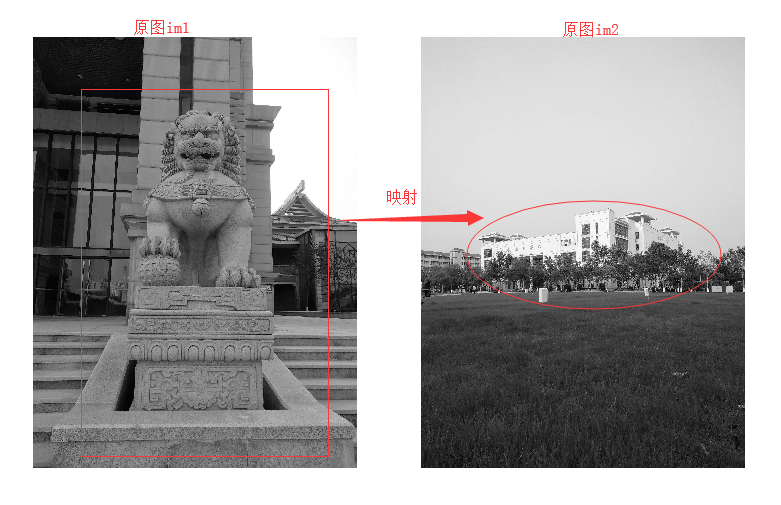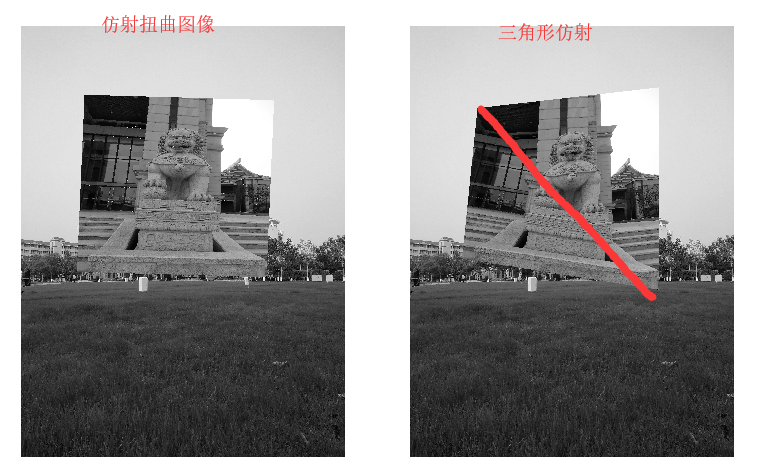1.仿射扭曲就是讲图像或者图像的一部分放置在另一幅图像中，使得它们能够和指定的区域或者标记物对齐。在函数image_in_image()中，函数的输入参数是两幅图像和一个坐标。该坐标为将第一幅图像放置到第二幅图像中的角点坐标：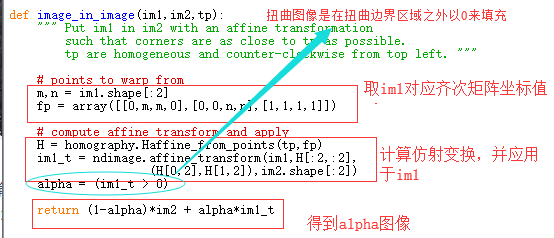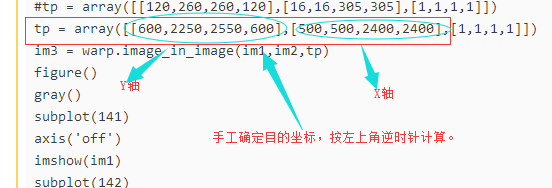## （三）遇到的问题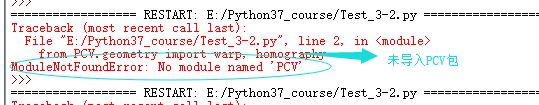cd PCV
python setup.py install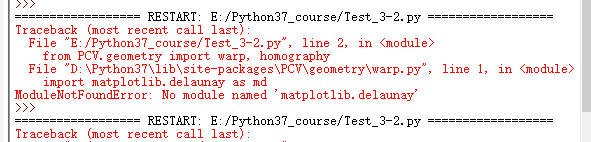2019-08-30 17:38:03 l2181265 阅读数 355
• ###### MATLAB图像处理

全面系统的学习MATLAB在图像处理中的应用

19901 人正在学习 去看看 魏伟

DCN论文的贡献是提出Deformable Conv。

1、Deformable Conv
2、Deformable ROI pooling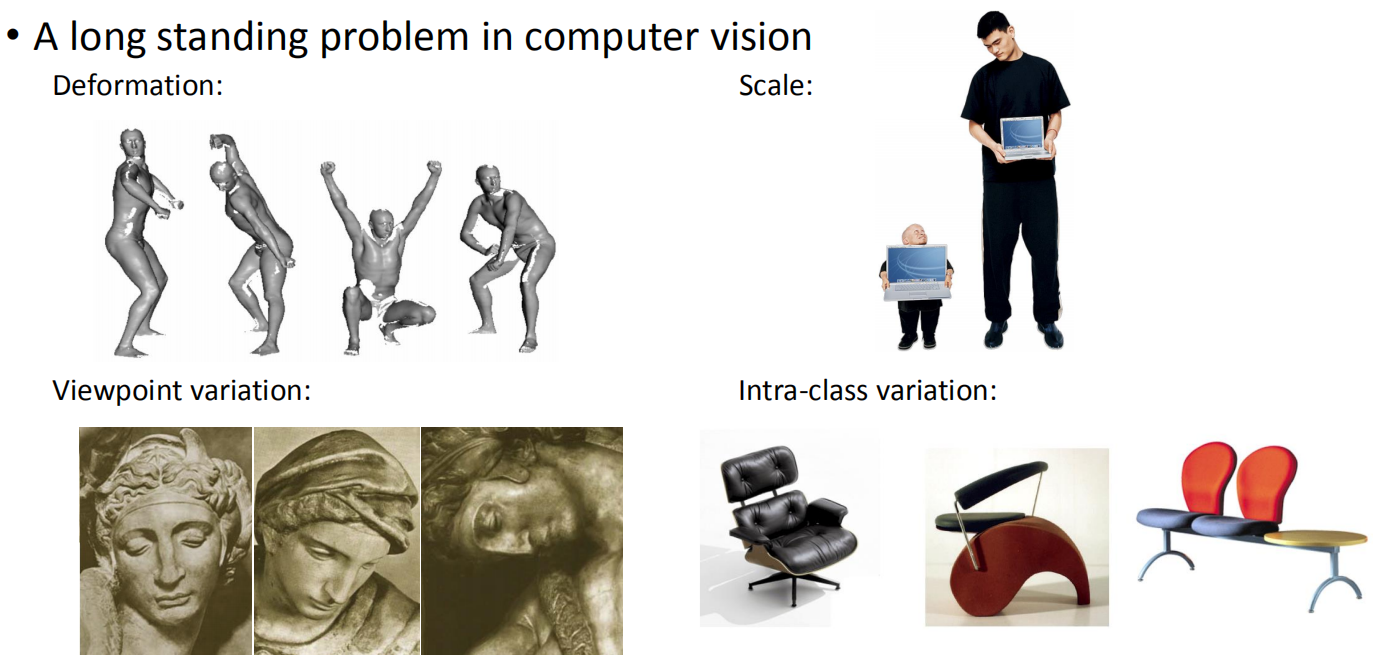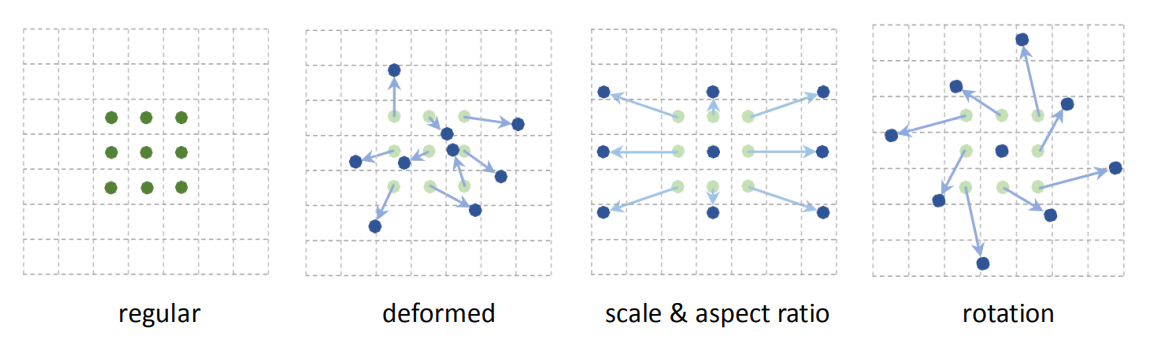Deformable Conv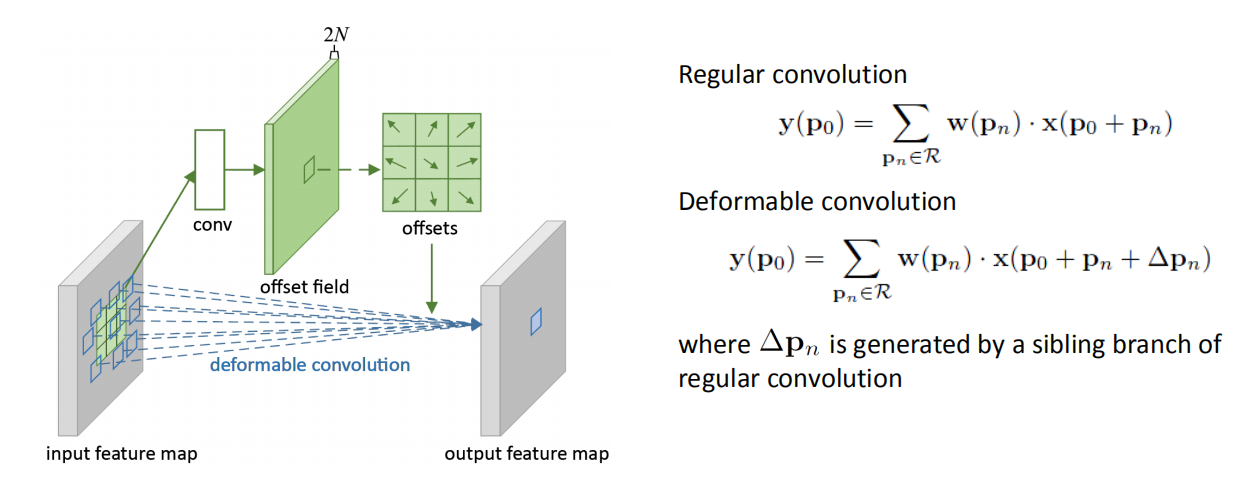w(pn)是网络学习的参数（权重），即卷积核；x(p0+pn)是像素p0+pn的像素值，如下图，我们使用3×3的卷积核，pn是p0附近的8个坐标。
Δpn是基于经典卷积的偏移量，是需要输入数据通过网络学习而得的。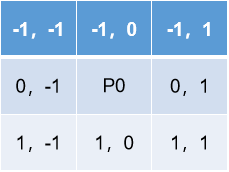Deformable ROI pooling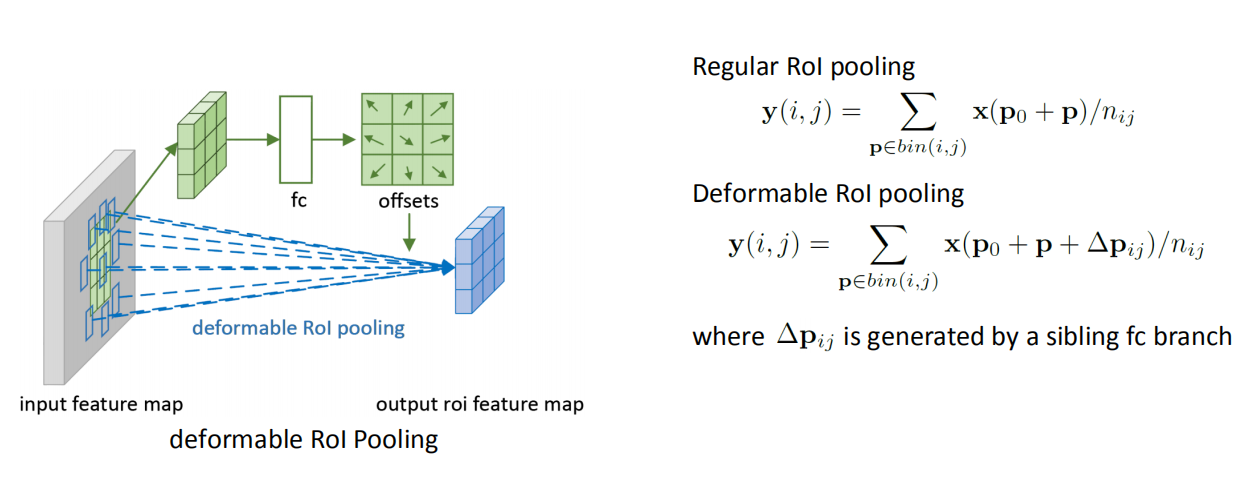Fast RCNN取得是max pooling，而本文中是取得mean pooling。这里也是通过学习它的偏移量，使ROI映射的小窗口更接近于真实的目标。

Deformable Conv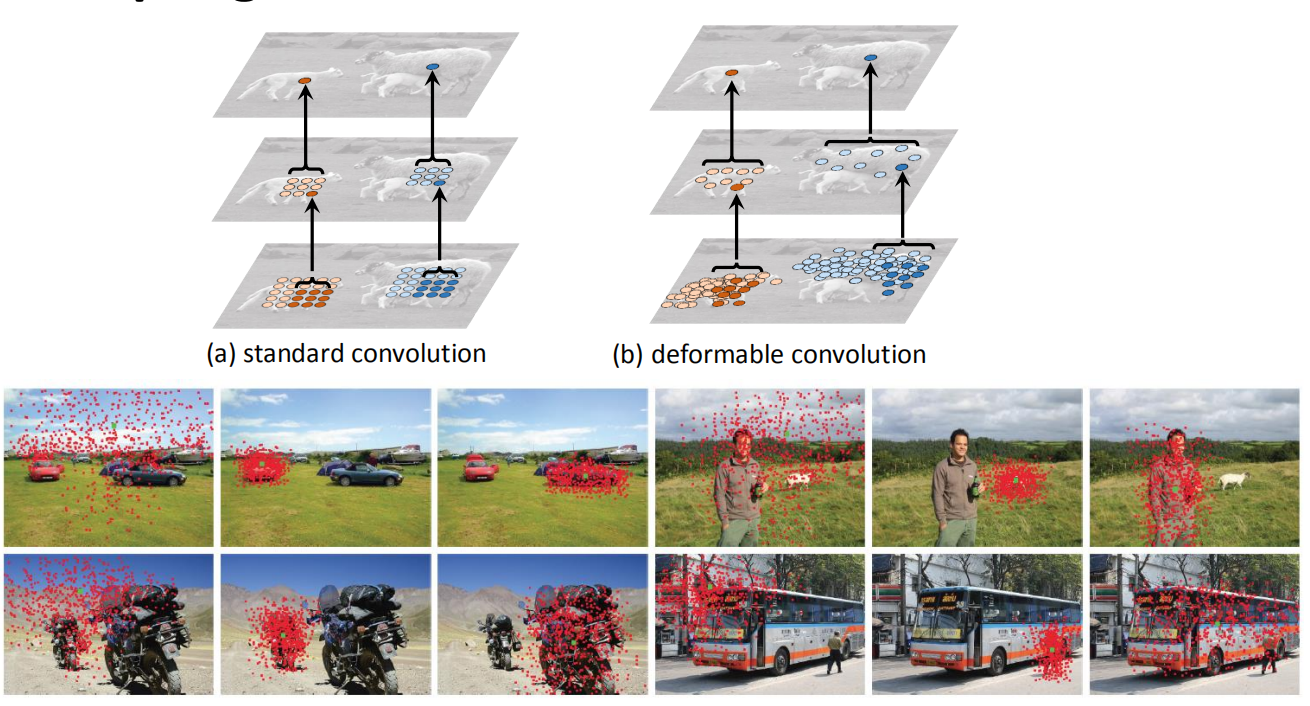Deformable ROI pooling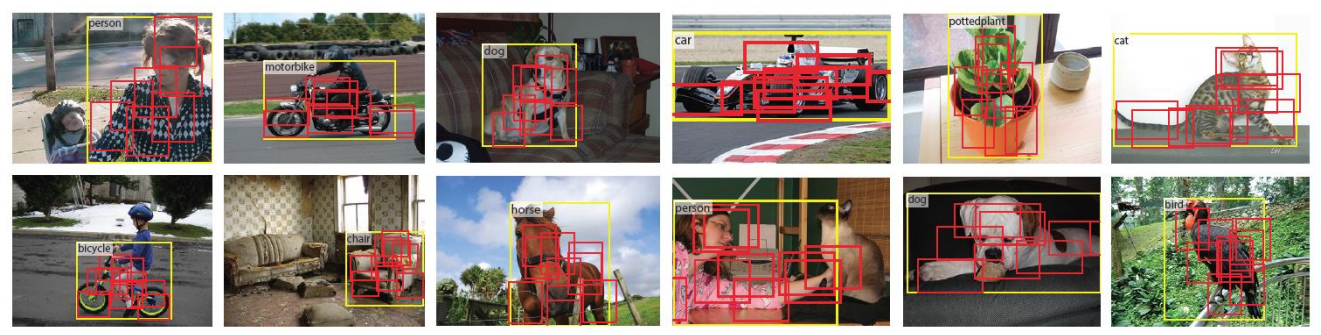2017-12-13 11:27:59 lingzidong 阅读数 276
• ###### MATLAB图像处理

全面系统的学习MATLAB在图像处理中的应用

19901 人正在学习 去看看 魏伟

# 总览

## 图像的缩放：尺度变换

cv2.INTER_AREA : 最近邻插值，把一个点的像素值直接设置为这个点最近的点的像素，但是这个方法会让图像出现很多色块
cv2.INTER_LINEAR ： 双线性插值，这里使用了一维下的线性插值算法在二维上的推广，一维上的插值算法就是在两点之间填上一条直线，二维中只要4个方程对上下左右四个像素解方程就行
cv2.INTER_CUBIC：立方插值是在双线性插值算法的基础上进行改进，除了考虑函数值以外还添加了变化率的估量，只不过速度很慢就是了。

import cv2
import numpy as np

# 按照倍数缩放

res1 = cv2.resize(img,(0,0),fx = 1.3,fy = 1.3,interpolation=cv2.INTER_CUBIC)

# 按照大小进行变化
res2 = cv2.resize(img,(500,500),interpolation=cv2.INTER_AREA)

cv2.imshow("out1",res1)
cv2.imshow("out2",res2)

cv2.waitKey(0)

## 图像的旋转

import cv2
import numpy as np

# 旋转90°

row,col,ch = img.shape
M = cv2.getRotationMatrix2D(((col/2),(row/2)),90,1)
# 参数分别是中心点，角度，缩放尺度
res1 = cv2.warpAffine(img,M,(row,col))

cv2.imshow("out1",res1)

cv2.waitKey(0)

## 仿射变换和透视变换：

import cv2
import numpy as np

# 通过三个点的相对位置来确定整个图像

row,col,ch = img.shape

pts1 = np.float32([[0,0],[0,col],[row,0]])
pts2 = np.float32([[0,0],[100,col],[row,50]])
M1 = cv2.getAffineTransform(pts1,pts2)
res1 = cv2.warpAffine(img,M1,(row,col))

pts3 = np.float32([[0,0],[0,col],[row,0],[row,col]])
pts4 = np.float32([[10,10],[10,col-10],[row-10,10],[row-10,col-10]])
M2 = cv2.getPerspectiveTransform(pts3,pts4)

res2 = cv2.warpPerspective(img,M2,(row,col))

cv2.imshow("out1",res1)
cv2.imshow("out2",res2)
cv2.waitKey(0)